# Energy Changes

## Energy Transfer in Reactions

Different chemicals contain different amount of energy. This energy is stored __in the __bonds between atoms. If the total energy of the products is less than the total energy of the reactants, then energy has been lost during reaction. The energy had been lost through heat (or even sometimes light and noise energy). If the total energy of the products is more than the total energy of the reactants, then energy has been gained during reaction. The extra energy has usually come from heat or light energy being put into a reaction (for example, when you use a bunsen burner to heat something up).

## Exothermic and Endothermic

What you need to know:

The difference between exothermic and endothermic reactions.

An exothermic reaction is when energy is lost to the surroundings. This usually results in a rise in temperature when two things react.

An endothermic reaction is when energy is taken from the surroundings. This usually results in a drop in temperature when two things react.

The amount of energy transfer is proportional to the temperature change of a reaction. You can test if a reaction is exothermic or endothermic by using the equipment below: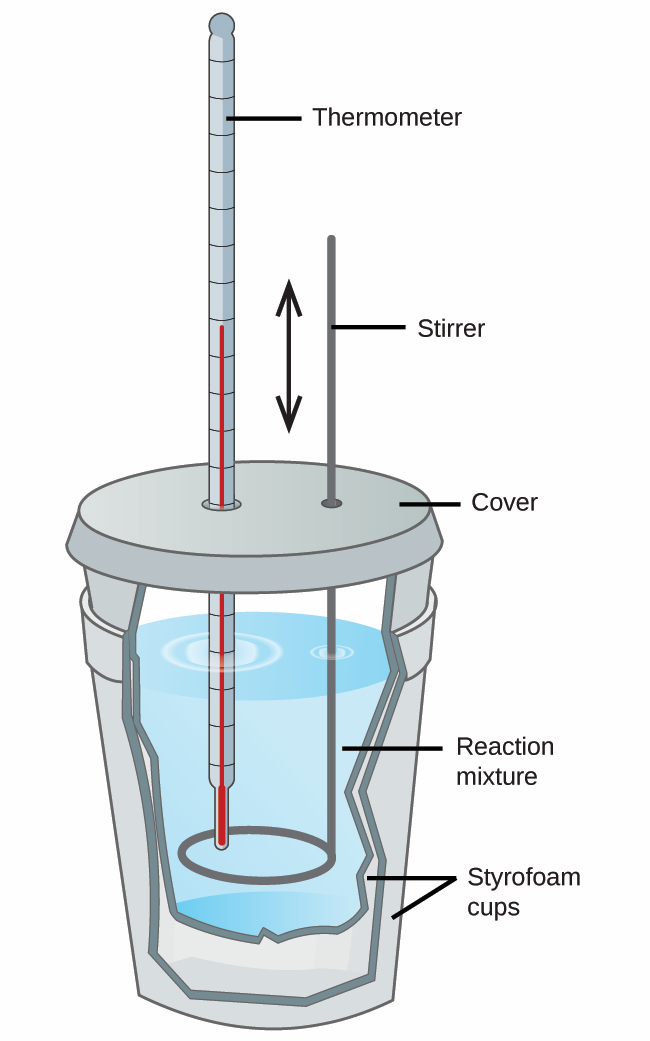Note: the cup needs to be insulated for best results, this means that less of the energy leaves the cup so you get a better result.

## Reaction Profiles

What you need to know:

What a reaction profile is and how to analyse a reaction profile.

Reaction profiles are just graphs of the energy change thats happening in a reaction.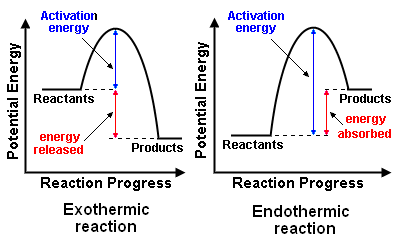There are three main things you need to know about these:

1. The overall energy change of the reaction - this is the difference between the reactants energy and the products energy. If the reactants have more energy than the products, energy has been released. If the reactants have less energy than the products, energy has been absorbed by the particles.
2. Whether the reaction is exothermic or endothermic - If the reactants have more energy than the products, energy has been released and this is EXOTHERMIC. If the reactants have less energy than the products, energy has been absorbed by the particles and this is ENDOTHERMIC.
3. The activation energy - This is the minimum amount of energy that the particles need in order to react with each other. Energy usually needs to be put in in order to break the bonds and start the reaction. The activation energy is labelled on the graphs above.

## Energy in Reactions

What you need to know:

1. How to analyse reaction profiles.
2. How the release/intake of energy depends on if bonds are being make/broken.
3. Energy is measured in Joules (J)
4. Energy lost/gained in a reaction is usually measured in kJ/mol. Since the amount of energy transfer depends on how much reactant there was.

Energy of bonds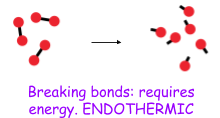Forming bonds gives out energy, so this is exothermic.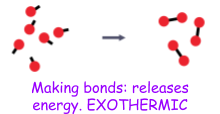Whether a reaction is endothermic or exothermic depends on whether there were more bonds being broken or more bonds being formed.﻿

## Energy Calcuations

__What you need to know: __

Not all bonds have the same strength, it will require more energy to break some bonds than it will to break others.

You may have to work out if something is exothermic or endothermic just by looking at the bond energies.

__To do this you need to: __

1. Draw out the displayed formulas for all of the reactants in the reaction.
2. Work out how much energy would be needed to break all of these bonds
3. Draw out all the displayed formulas for all of the products in the reaction.
4. Work out how much energy would be releases when making all of these bonds
5. Use the formula: Energy change = energy of bond breaking - energy of bond making.
6. If the energy change is a negative number, the reaction is exothermic. If the energy change is a positive number, the reaction is endothermic.

## Cells

What you need to know:

What a chemical cell is and how they work.

A cell is a piece of equipment that contains two chemicals that react together to create an electricity. It contains two electrodes and an electrolyte (the liquid that contains the chemicals). The electrodes need to be able to conduct electricity so these are made of metal. If the two electrodes are connected by a conducting wire, the electrons can flow and a current (electricity) is produced. This can be used to power things, like torches!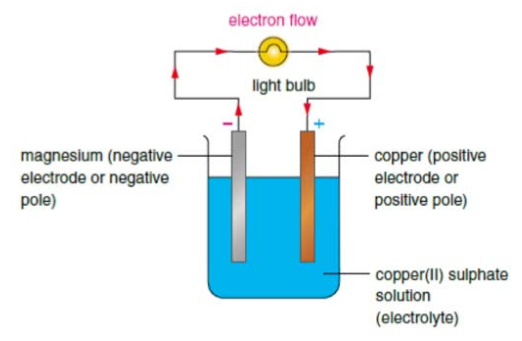## Batteries

What you need to know:

What a chemical battery is and how they work.

A battery is simply when two or more cells are put together in series. A battery can be rechargeable or non rechargeable. This depends on whether the reaction that takes place is reversible or not.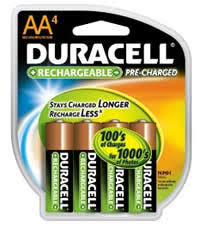1. If the reaction is reversible, the batteries can be recharged and used again
2. If the reaction is irreversible, the batteries can only be used once

## Fuel Cells

__What you need to know: __

What a fuel cell is and how one works.

A fuel cell is a device that uses fuel and oxygen (from the air) to produce an electric current. This happens in cars! Oxygen (from the air) reacts with hydrogen (from the fuel) to produce water.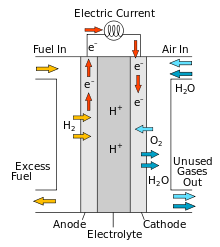1. At the negative electrode, the hydrogen from the fuel loses electrons to produce H+ ions. These H+ions move to the negative electrode. This is oxidation.
2. At the positive electrode, the oxygen from the air gain electrons and react with the H+ ions to produce water. These H+ions move to the negative electrode. This is reduction.
3. The electrons flow through the wire from the negative electrode to the positive electrode. A device that needs to be powered can be placed between the wires so that the electrons pass through on their way to the positive electrode.